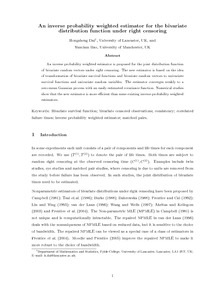# An inverse probability weighted estimator for the bivariate distribution function under right censoring

Dai, H and Bao, Y (2009) 'An inverse probability weighted estimator for the bivariate distribution function under right censoring.' Statistics and Probability Letters, 79 (16). 1789 - 1797. ISSN 0167-7152Preview
Text
DAIBivariate.pdf - Accepted Version

Download (198kB) | Preview

## Abstract

An inverse probability weighted estimator is proposed for the joint distribution function of bivariate random vectors under right censoring. The new estimator is based on the idea of transformation of bivariate survival functions and bivariate random vectors to univariate survival functions and univariate random variables. The estimator converges weakly to a zero-mean Gaussian process with an easily estimated covariance function. Numerical studies show that the new estimator is more efficient than some existing inverse probability weighted estimators. © 2009 Elsevier B.V. All rights reserved.

Item Type: Article Q Science > QA Mathematics Faculty of Science and Health > Mathematical Sciences, Department of Jim Jamieson 12 Feb 2013 08:08 02 Sep 2019 21:15 http://repository.essex.ac.uk/id/eprint/5494

### Actions (login required)View Item